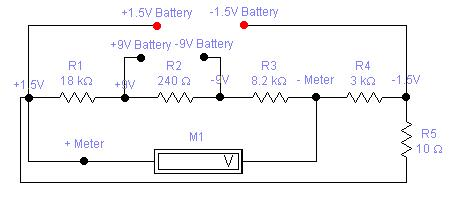Battery Tester for 1.5 and 9V
by Matthew B.Parts List:
R1 = 18K
R2 = 240 Ohm
R3 = 8.2K
R4 = 3K
R5 = 10 Ohm
M1 = Panel Meter (Anyone will work)

Design Considerations:
You may have experiment with the values of R3 and R4 to get an accurate reading from the meter. Every meter is different, so a little bit of playing with the resistor values is required.

Try using a variable resistor in place of R3 & R4 to get a value of resistance that works.